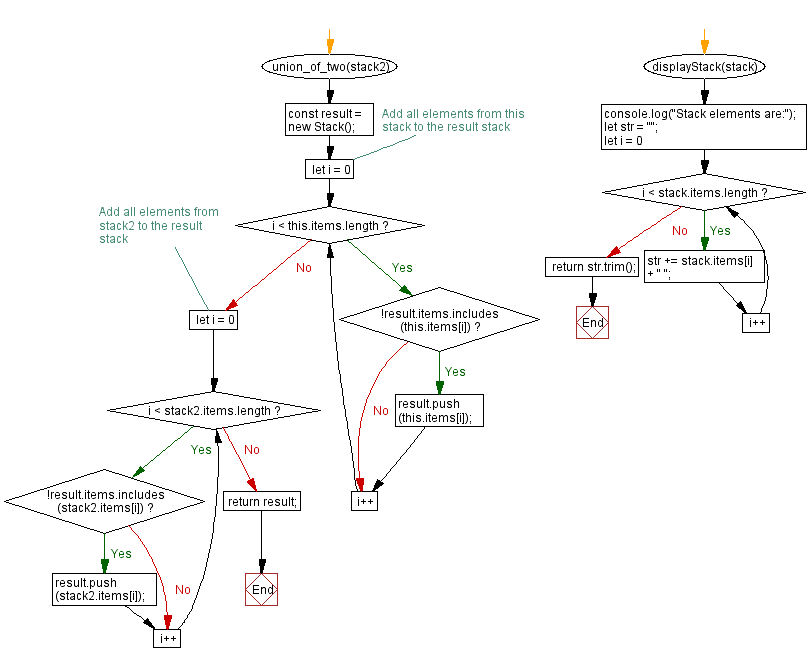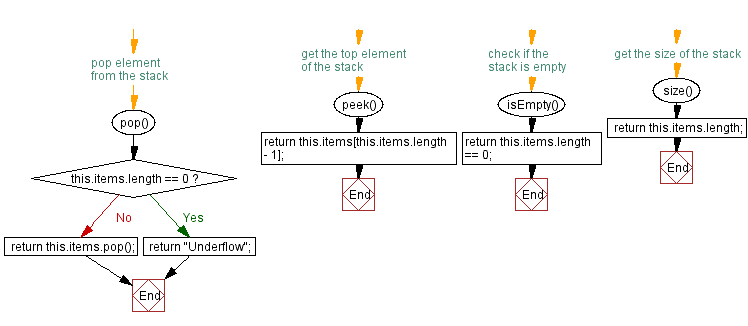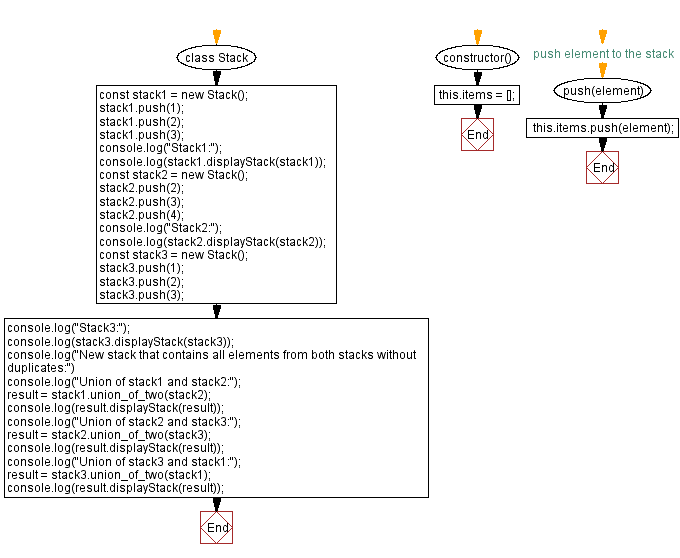# JavaScript Exercises: Elements from both stacks without duplicates

## JavaScript Stack: Exercise-30 with Solution

Write a JavaScript program that implements a stack and creates a new stack that contains all elements from both stacks without duplicates.

Sample Solution:

JavaScript Code:

``````class Stack {
constructor() {
this.items = [];
}

// push element to the stack
push(element) {
this.items.push(element);
}

// pop element from the stack
pop() {
if (this.items.length == 0) {
return "Underflow";
}
return this.items.pop();
}

// get the top element of the stack
peek() {
return this.items[this.items.length - 1];
}

// check if the stack is empty
isEmpty() {
return this.items.length == 0;
}

// get the size of the stack
size() {
return this.items.length;
}

union_of_two(stack2) {
const result = new Stack();

// Add all elements from this stack to the result stack
for (let i = 0; i < this.items.length; i++) {
if (!result.items.includes(this.items[i])) {
result.push(this.items[i]);
}
}

// Add all elements from stack2 to the result stack
for (let i = 0; i < stack2.items.length; i++) {
if (!result.items.includes(stack2.items[i])) {
result.push(stack2.items[i]);
}
}
return result;
}
displayStack(stack) {
console.log("Stack elements are:");
let str = "";
for (let i = 0; i < stack.items.length; i++)
str += stack.items[i] + " ";
return str.trim();
}
}
const stack1 = new Stack();
stack1.push(1);
stack1.push(2);
stack1.push(3);
console.log("Stack1:");
console.log(stack1.displayStack(stack1));
const stack2 = new Stack();
stack2.push(2);
stack2.push(3);
stack2.push(4);
console.log("Stack2:");
console.log(stack2.displayStack(stack2));
const stack3 = new Stack();
stack3.push(1);
stack3.push(2);
stack3.push(3);
console.log("Stack3:");
console.log(stack3.displayStack(stack3));
console.log("New stack that contains all elements from both stacks without duplicates:")
console.log("Union of stack1 and stack2:");
result = stack1.union_of_two(stack2);
console.log(result.displayStack(result));
console.log("Union of stack2 and stack3:");
result = stack2.union_of_two(stack3);
console.log(result.displayStack(result));
console.log("Union of stack3 and stack1:");
result = stack3.union_of_two(stack1);
console.log(result.displayStack(result));
```
```

Sample Output:

```Stack1:
Stack elements are:
1 2 3
Stack2:
Stack elements are:
2 3 4
Stack3:
Stack elements are:
1 2 3
New stack that contains all elements from both stacks without duplicates:
Union of stack1 and stack2:
Stack elements are:
1 2 3 4
Union of stack2 and stack3:
Stack elements are:
2 3 4 1
Union of stack3 and stack1:
Stack elements are:
1 2 3
```

Flowchart:Live Demo:

See the Pen javascript-stack-exercise-30 by w3resource (@w3resource) on CodePen.

Improve this sample solution and post your code through Disqus

Stack Previous: Find elements that are in both stacks.
Stack Exercises Next: Symmetric difference of two stacks.

What is the difficulty level of this exercise?

Test your Programming skills with w3resource's quiz.

﻿